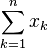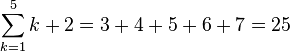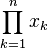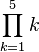# List of mathematical symbols facts for kids

Kids Encyclopedia Facts
(Redirected from Table of mathematical symbols)

The following list contains some of the most notable symbols in mathematics. Please note that these symbols may have alternate meanings in different contexts.

Symbol Name Read as Meaning Example
=
equality equals, is equal to If x=y, x and y represent the same value or thing. 2+3=5
definition is defined as If x≡y, x is defined as another name of y (a+b)2≡a2+2ab+b2
approximately equal is approximately equal to If x≈y, x and y are almost equal. √2≈1.41
inequation does not equal, is not equal to If x≠y, x and y do not represent the same value or thing. 1+1≠3
<
strict inequality
is less than If x<y, x is less than y. 4<5
>
is greater than If x>y, x is greater than y. 3>2
is much less than If x≪y, x is much less than y. 1≪999999999
is much greater than If x≫y, x is much greater than y. 88979808≫0.001
inequality
is less than or equal to If x≤y, x is less than or equal to y. 5≤6 and 5≤5
is greater than or equal to If x≥y, x is greater than or equal to y. 2≥1 and 2≥2
proportionality is proportional to If x∝y, then y=kx for some constant k. If y=4x then y∝x and x∝y
+
addition plus x+y is the sum of x and y. 2+3=5
-
subtraction minus x-y is the subtraction of y from x 5-3=2
×
multiplication times x×y is the multiplication of x by y 4×5=20
·
x·y is the multiplication of x by y 4·5=20
÷
division divided by x÷y or x/y is the division of x by y 20÷4=5 and 20/4=5
/
20/4=5
±
plus-minus plus or minus x±y means both x+y and x-y The equation 3±√9 has two solutions, 0 and 6.
minus-plus minus or plus 4±(3∓5) means both 4+(3-5) and 4-(3+5) 6∓(1±3)=2 or 4
square root square root √x is a number whose square is x. √4=2 or -2
summation sum over … from … to … of, sigma$\sum_{k=1}^{n}{x_k}$ is the same as x1+x2+x3+xk$\sum_{k=1}^{5}{k+2}=3+4+5+6+7=25$
multiplication product over … from … to … of$\prod_{k=1}^{n}{x_k}$ is the same as x1×x2×x3×xk$\prod_{k=1}^{5}{k}$=1×2×3×4×5=120
!
factorial factorial n! is the product 1×2×3...×n 5!=1×2×3×4×5=120
material implication implies A⇒B means that if A is true, B must also be true, but if A is false, B is unknown. x=3⇒x2=9, but x2=9⇒x=3 is false, because x could also be -3.
material equivalence if and only if If A is true, B is true and if A is false, B is false. x=y+1⇔x-1=y
|…|
absolute value absolute value of |x| is the distance along the real line (or across the complex plane) between x and zero |5|=5 and |-5|=5
||
parallel is parallel to If A||B then A and B are parallel
perpendicular is perpendicular to If A⊥B then A is perpendicular to B
congruence is congruent to If A≅B then shape A is congruent to shape B (has the same measurements)
φ
golden ratio golden ratio The golden ratio is an irrational number equal to (1+√5)÷2 or approximately 1.6180339887.
infinity infinity ∞ is a number greater than every real number.
set membership is an element of a∈S means that a is an element of the set S 3.5∈ℝ, 1∈ℕ, 1+i∈ℂ
is not an element of a∉S means that a is not an element of the set S 2.1∉ℕ, 1+i∉ℝ
{,}
Set brackets the set of {a,b,c} is the set consisting of a, b, and c ℕ={0,1,2,3,4,5...}
Natural numbers N ℕ denotes the set of natural numbers {0,1,2,3,4,5...}
Integers Z ℤ denotes the set of integers (-3,-2,-1,0,1,2,3...)
Rational numbers Q ℚ denotes the set of rational numbers (numbers that can be written as a fraction a/b where a∈ℤ, b∈ℕ) 8.323∈ℚ, 7∈ℚ, π∉ℚ
Real numbers R ℝ denotes the set of real numbers π∈ℝ, 7∈ℝ, √(-1)∉ℝ
Complex numbers C ℂ denotes the set of complex numbers √(-1)∈ℂ
Mean bar, overbar x̄ is the mean (average) of xi if x={1,2,3} then x̄=2
complex conjugate the complex conjugate of x If x=a + bi, then x̄=a - bi where i=√(-1) x=-4 + 5.3i, x̄=-4 - 5.3i

## Related pagesList of mathematical symbols Facts for Kids. Kiddle Encyclopedia.